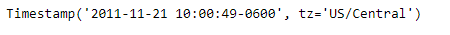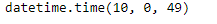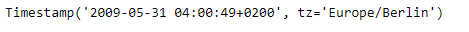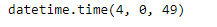# Python | Pandas Timestamp.time

Python is a great language for doing data analysis, primarily because of the fantastic ecosystem of data-centric python packages. Pandas is one of those packages and makes importing and analyzing data much easier.

Pandas` Timestamp.time()` function return time object with same time but with tzinfo equal to None.

Syntax :Timestamp.time()

Parameters : None

Return : datetime object

Example #1: Use `Timestamp.time()` function to return the time from the given Timestamp object.

 `# importing pandas as pd ` `import` `pandas as pd ` ` `  `# Create the Timestamp object ` `ts ``=` `pd.Timestamp(year ``=` `2011``,  month ``=` `11``, day ``=` `21``, ` `           ``hour ``=` `10``, second ``=` `49``, tz ``=` `'US/Central'``) ` ` `  `# Print the Timestamp object ` `print``(ts) `

Output :Now we will use the `Timestamp.time()` function to return the time

 `# return time ` `ts.time() `

Output :As we can see in the output, the `Timestamp.time()` function has returned the time of the given Timestamp object. Notice the given object is not aware of the timezone.

Example #2: Use `Timestamp.time()` function to return the time from the given Timestamp object.

 `# importing pandas as pd ` `import` `pandas as pd ` ` `  `# Create the Timestamp object ` `ts ``=` `pd.Timestamp(year ``=` `2009``, month ``=` `5``, day ``=` `31``, ` `       ``hour ``=` `4``, second ``=` `49``, tz ``=` `'Europe/Berlin'``) ` ` `  `# Print the Timestamp object ` `print``(ts) `

Output :Now we will use the `Timestamp.time()` function to return the time

 `# return time ` `ts.time() `

Output :As we can see in the output, the `Timestamp.time()` function has returned the time of the given Timestamp object. Notice the given object is not aware of the timezone.

Whether you're preparing for your first job interview or aiming to upskill in this ever-evolving tech landscape, GeeksforGeeks Courses are your key to success. We provide top-quality content at affordable prices, all geared towards accelerating your growth in a time-bound manner. Join the millions we've already empowered, and we're here to do the same for you. Don't miss out - check it out now!

Previous
Next